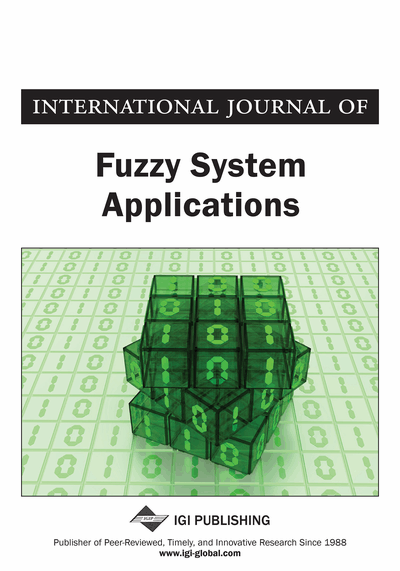# Eigenvalue of Intuitionistic Fuzzy Matrices Over Distributive Lattice

Ali Ebrahimnejad (Department of Mathematics, Qaemshahr Branch, Islamic Azad University, Qaemshar, Iran), Amal Kumar Adak (Department of Applied Mathematics, Vidyasagar University, Midnapore, India), and Ezzatallah Baloui Jamkhaneh (Department of Statistics, Qaemshahr Branch, Islamic Azad University, Qaemshar, Iran)
DOI: 10.4018/IJFSA.2019010101
Available
\$29.50
No Current Special Offers

## Abstract

In this article, the concepts of intuitionistic fuzzy complete and complete distributive lattice are introduced and the relative pseudocomplement relation of intuitionistic fuzzy sets is defined. The concepts of intuitionistic fuzzy eigenvalue and eigenvector of an intuitionistic fuzzy matrixes are presented and proved that the set of intuitionistic fuzzy eigenvectors of a given intuitionistic fuzzy eigenvalue form an intuitionistic fuzzy subspace. Also, the authors obtain an intuitionistic fuzzy maximum matrix of a given intuitionistic fuzzy eigenvalue and eigenvector and give some properties of an intuitionistic fuzzy maximum matrix. Finally, the invariant of an intuitionistic fuzzy matrix over a distributive lattice is given with some properties.
Article Preview
Top

## 1. Introduction

Atanassov (1986) introduced the concept of intuitionistic fuzzy sets (IFSs). After that lot of reseach works done by several researchers on the field of IFS (Li, 2014; Robinson and Amirtharaj, 2015; Goyal et al., 2016; Li and Wan, 2017; Nan, Li & An, 2017; Nan et al., 2017; Bhaumika et al., 2017; Ren et al., 2017; Yu et al., 2017, Aikhuele and Turan, 2017; Ebrahimnejad and Verdegay, 2016; Ebrahimnejad and Verdegay, 2017). By the concept of IFSs, first time Pal (2001) introduced intuitionistic fuzzy determinant. Later on, Pal et al. (2002) and Shyamal and Pal (2002) introduced intuitionistic fuzzy matrices and determined distance between intuitionistic fuzzy matrices. Bhowmik and Pal (2003, 2008) introduced some results on intuitionistic fuzzy matrices and intuitionistic circulant fuzzy matrices and generalized intuitionistic fuzzy matrices.

The eigenvector-eigenvalues problem of matrices over distributive lattices have appeared firstly in the work of Rutherford (1965). He showed that given an eigenvector of a matrix over Boolean algebra the eigenvalues of the matrix with the given eigenvector vector form an interval in this algebra and give an explicit formula for this interval. Kirkland and Pullman (1992) presented a convenient way to construct spanning set of minimum cardinality for each eigenspace in the case of finite Boolean algebras. Sanches (1978) presented a way to find the maximum standard eigenvector of a given fuzzy matrix. Cechlarova (1992) characterized the standard eigenvectors by means of the associated graph of a matrix over the Bottleneck algebra. Gavalec (2002) studied the set of all increasing eigenvectors of a matrix over a max-min algebra.

The fuzzy eigenvector and eigenvalue of a fuzzy matrix play a center role in mathematics and engineering. Fuzzy eigenvalues were first studied in Buckley (1990) to analyse input-output of systems. Massa (2008) determined both the fuzzy eigenvalues and eigenvectors of a finite model defined with fuzzy parameters. Alevizos et al. (2007) converted the fuzzy eigenvalue problem into an ordinary one in order to study correspondence data. To produce a fuzzy eigenvector weight estimate, Wang and Chin (2006) used the ordinary eigenvector through the solution of a linear programming model. Our aim in this study is to introduce the intuitionistic fuzzy eigenvalue and eigenvector for an intuitionistic fuzzy martix (IFM) over the complete and complete intuitionistic fuzzy distributive lattice.

The structure of this paper is organized as follows. In Section 2, the preliminaries and some definitions are given. In Section 3, the concept of pseudocomplement relation is introduced. In Section 4, intuitionistic fuzzy eigenvalue and eigenvector are defined and the maximum intuitionistic fuzzy eigenvector of a given intuitionistic fuzzy matrix for a given intuitionistic fuzzy eigenvalue are obtained. Also, some properties of maximum intuitionistic fuzzy matrix from the set of intuitionistic fuzzy matrices with a given intuitionistic fuzzy eigenvalue and eigenvector are studied. In Section 5, invariant intuitionistic fuzzy matrix is defined and some properties of invariant intuitionistic fuzzy matrix are presented. Conclusions are made in Section 6.

Top

## 2. Preliminaries

In this section, we first give some preliminaries, definitions of IFSs and IFMs and then present some algebraic operations of IFMs and different types of IFMs (Adak and Bhowmik 2011, Adak et al. 2011a, b; Adak et al. 2012a, b, 2013).

## Complete Article List

Search this Journal:
Reset
Volume 12: 1 Issue (2023)
Volume 11: 4 Issues (2022)
Volume 10: 4 Issues (2021)
Volume 9: 4 Issues (2020)
Volume 8: 4 Issues (2019)
Volume 7: 4 Issues (2018)
Volume 6: 4 Issues (2017)
Volume 5: 4 Issues (2016)
Volume 4: 4 Issues (2015)
Volume 3: 4 Issues (2013)
Volume 2: 4 Issues (2012)
Volume 1: 4 Issues (2011)
View Complete Journal Contents Listing